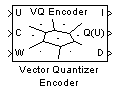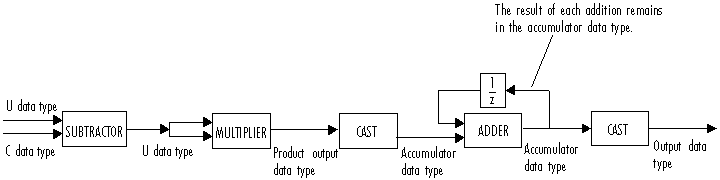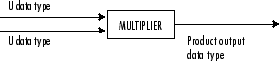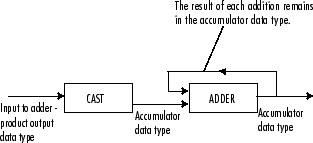# Vector Quantizer Encoder

For given input, find index of nearest codeword based on Euclidean or weighted Euclidean distance measure

## Library

Quantizers

`dspquant2`

•## Description

The Vector Quantizer Encoder block compares each input column vector to the codeword vectors in the codebook matrix. Each column of this codebook matrix is a codeword. The block finds the codeword vector nearest to the input column vector and returns its zero-based index. This block supports real floating-point and fixed-point signals on all input ports.

The block finds the nearest codeword by calculating the distortion. The block uses two methods for calculating distortion: Euclidean squared error (unweighted) and weighted Euclidean squared error. Consider the codebook, $CB=\left[\begin{array}{cccc}C{W}_{1}& C{W}_{2}& ...& C{W}_{N}\end{array}\right]$. This codebook has N codewords; each codeword has k elements. The i-th codeword is defined as a column vector, $C{W}_{i}=\left[\begin{array}{cccc}{a}_{1i}& {a}_{2i}& ...& {a}_{ki}\end{array}\right]$. The multichannel input has M columns and is defined as $U=\left[\begin{array}{cccc}{U}_{1}& {U}_{2}& ...& {U}_{M}\end{array}\right]$, where the p-th input column vector is ${U}_{p}={\left[\begin{array}{cccc}{u}_{1p}& {u}_{2p}& ...& {u}_{kp}\end{array}\right]}^{\prime }$. The squared error (unweighted) is calculated using the equation

`$D=\sum _{j=1}^{k}{\left({a}_{ji}-{u}_{jp}\right)}^{2}$`

The weighted squared error is calculated using the equation

`$D=\sum _{j=1}^{k}{w}_{j}{\left({a}_{ji}-{u}_{jp}\right)}^{2}$`

where the weighting factor is defined as $W=\left[\begin{array}{cccc}{w}_{1}& {w}_{2}& ...& {w}_{k}\end{array}\right]$. The index of the codeword that is associated with the minimum distortion is assigned to the input column vector.

You can select how you want to enter the codebook values using the Source of codebook parameter. When you select ```Specify via dialog```, you can type the codebook values into the block parameters dialog box. Select `Input port` and port C appears on the block. The block uses the input to port C as the Codebook parameter.

The Codebook parameter is an k-by-N matrix of values, where k ≥ 1 and N ≥ 1. Each input column vector is compared to this codebook. Each column of the codebook matrix is a codeword, and each codeword has an index value. The first codeword vector corresponds to an index value of 0, the second codeword vector corresponds to an index value of 1, and so on. The codeword vectors must have the same number of rows as the input, U.

For the Distortion measure parameter, select ```Squared error``` when you want the block to calculate the distortion by evaluating the Euclidean distance between the input column vector and each codeword in the codebook. Select `Weighted squared error` when you want to use a weighting factor to emphasize or deemphasize certain input values.

For the Source of weighting factor parameter, select `Specify via dialog` to enter a weighting factor vector in the dialog box. Choose `Input port` to specify the weighting factor using port W.

Use the Weighting factor parameter to emphasize or deemphasize certain input values when calculating the distortion measure. For example, consider the p-th input column vector, ${U}_{p}$, as previously defined. When you want to neglect the effect of the first element of this vector, enter `[0 1 1 ... 1]` as the Weighting factor parameter. This weighting factor is used to calculate the weighted squared error using the equation

`$D=\sum _{j=1}^{k}{w}_{j}{\left({a}_{ji}-{u}_{jp}\right)}^{2}$`

Because of the weighting factor used in this example, the weighted squared error is not affected by the first element of the input matrix. Therefore, the first element of the input column vector no longer impacts the choice of index value output by the Vector Quantizer Encoder block.

Use the Index output data type parameter to specify the data type of the index values output at port I. The data type of the index values can be `int8`, `uint8`, `int16`, `uint16`, `int32`, or `uint32`.

When an input vector is equidistant from two codewords, the block uses the Tie-breaking rule parameter to determine which index value the block chooses. When you want the input vector to be represented by the lower index valued codeword, select `Choose the lower index`. To represent the input column vector by the higher index valued codeword, select ```Choose the higher index```.

Select the Output codeword check box to output at port Q(U) the codeword vectors that correspond to each index value. When the input is a matrix, the corresponding codeword vectors are horizontally concatenated into a matrix.

Select the Output quantization error check box to output at port D the quantization error that results when the block represents the input column vector by its nearest codeword. When the input is a matrix, the quantization error values are horizontally concatenated.

The Vector Quantizer Encoder block accepts real floating-point and fixed-point inputs. For more information on the data types accepted by each port, see Data Type Support or Supported Data Types.

### Data Type Support

The input data values, codebook values, and weighting factor values are input to the block at ports U, C, and W, respectively. The data type of the input data values, codebook values, and weighting factor values can be `double`, `single`, or Fixed-point. The input data, codebook values, and weighting factor must be the same data type.

The outputs of the block are the index values, output codewords, and quantization error. Use the Index output data type parameter to specify the data type of the index output from the block at port I. The data type of the index can be `int8`, `uint8`, `int16`, `uint16`, `int32`, or `uint32`. The data type of the output codewords and the quantization error can be `double`, `single`, or Fixed-point. The block assigns the data type of the output codewords and the quantization error based on the data type of the input data.

### Fixed-Point Data Types

The following diagram shows the data types used within the Vector Quantizer Encoder block for fixed-point signals.You can set the product output, accumulator, and index output data types in the block dialog as discussed below.

## Dialog Box

### Main Tab

Source of codebook

Choose `Specify via dialog` to type the codebook values into the block parameters dialog box. Select `Input port` to specify the codebook values using the block's input port, C.

Codebook

Enter a k-by-N matrix of values, where 1 ≤ k and 1 ≤ N, to which your input column vector or matrix is compared. This parameter is visible if, from the Source of codebook list, you select ```Specify via dialog```.

Distortion measure

Select `Squared error` when you want the block to calculate the distortion by evaluating the Euclidean distance between the input column vector and each codeword in the codebook. Select `Weighted squared error` when you want the block to calculate the distortion by evaluating a weighted Euclidean distance using a weighting factor to emphasize or deemphasize certain input values.

Source of weighting factor

Select `Specify via dialog` to enter a value for the weighting factor in the dialog box. Choose ```Input port``` and specify the weighting factor using port W on the block. This parameter is visible if, for the Distortion measure parameter, you select ```Weighted squared error```.

Weighting factor

Enter a vector of values. This vector must have length equal to the number of rows of the input, U. This parameter is visible if, for the Source of weighting factor parameter, you select `Specify via dialog`.

Tie-breaking rule

Set this parameter to determine the behavior of the block when an input column vector is equidistant from two codewords. When you want the input column vector to be represented by the lower index valued codeword, select `Choose the lower index`. To represent the input column vector by the higher index valued codeword, select `Choose the higher index`.

Output codeword

Select this check box to output the codeword vectors nearest to the input column vectors.

Output quantization error

Select this check box to output the quantization error value that results when the block represents the input column vector by the nearest codeword.

Index output data type

Select `int8`, `uint8`, `int16`, `uint16`, `int32`, or `uint32 `as the data type of the index output at port I. To inherit the index output data type, select `Inherit via back propagation`.

### Data Types Tab

Rounding mode

Specify the rounding mode for fixed-point operations as one of the following:

• `Floor`

• `Ceiling`

• `Convergent`

• `Nearest`

• `Round`

• `Simplest`

• `Zero`

For more details, see rounding mode.

Overflow mode

When you select this parameter, the block saturates the result of its fixed-point operation. When you clear this parameter, the block wraps the result of its fixed-point operation. For details on `saturate` and `wrap`, see overflow mode for fixed-point operations.

Product outputAs depicted above, the output of the multiplier is placed into the product output data type and scaling. Use this parameter to specify how you would like to designate this product output word and fraction lengths.

• When you select `Same as input`, these characteristics match those of the input to the block.

• When you select ```Binary point scaling```, you can enter the word length and the fraction length of the product output, in bits.

AccumulatorAs depicted above, inputs to the accumulator are cast to the accumulator data type. The output of the adder remains in the accumulator data type as each element of the input is added to it. Use this parameter to specify how you would like to designate the accumulator word and fraction lengths.

• When you select ```Same as product output```, these characteristics match those of the product output.

• When you select `Same as input`, these characteristics match those of the input to the block.

• When you select ```Binary point scaling```, you can enter the word length and the fraction length of the accumulator, in bits.

## References

Gersho, A. and R. Gray. Vector Quantization and Signal Compression. Boston: Kluwer Academic Publishers, 1992.

## Supported Data Types

PortSupported Data Types

U

• Double-precision floating point

• Single-precision floating point

• Fixed point (signed only)

• 8-, 16-, and 32-bit signed integers

C

• Double-precision floating point

• Single-precision floating point

• Fixed point (signed only)

• 8-, 16-, and 32-bit signed integers

W

• Double-precision floating point

• Single-precision floating point

• Fixed point (signed only)

• 8-, 16-, and 32-bit signed integers

I

• 8-, 16-, and 32-bit signed integers

• 8-, 16-, and 32-bit unsigned integers

Q(U)

• Double-precision floating point

• Single-precision floating point

• Fixed point (signed only)

• 8-, 16-, and 32-bit signed integers

D

• Double-precision floating point

• Single-precision floating point

• Fixed point (signed only)

• 8-, 16-, and 32-bit signed integers